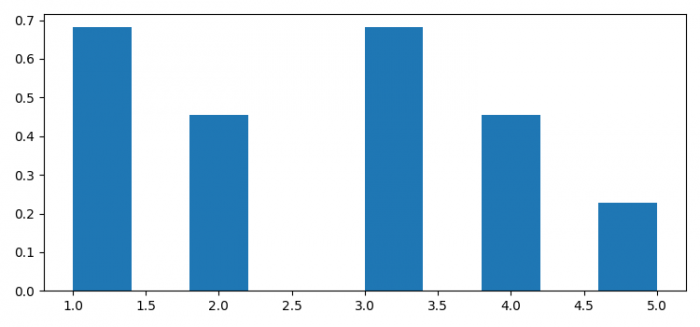# Vertical Histogram in Python and Matplotlib

MatplotlibPythonData Visualization

To plot vertical histogram in Python and Matplotlib, we can take the following steps −

• Set the figure size and adjust the padding between and around the subplots.
• Create a list of data points.
• Plot a histogram with vertical orientation.
• To display the figure, use show() method.

## Example

import matplotlib.pyplot as plt

plt.rcParams["figure.figsize"] = [7.50, 3.50]
plt.rcParams["figure.autolayout"] = True

x = [1, 2, 3, 1, 2, 3, 4, 1, 3, 4, 5]

plt.hist(x, orientation="vertical")

plt.show()

## Output